# Function

For linear function f(x) = ax + ​​b ‬ is f(14)=179; f(15)=154. Calculate m, if f(m) = 2019 .

Correct result:

m =  -59.6

#### Solution: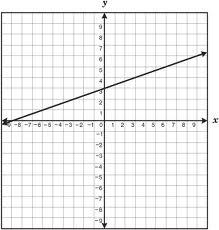We would be pleased if you find an error in the word problem, spelling mistakes, or inaccuracies and send it to us. Thank you!Tips to related online calculators
Do you have a linear equation or system of equations and looking for its solution? Or do you have quadratic equation?

## Next similar math problems:

• Linsys2Solve two equations with two unknowns: 400x+120y=147.2 350x+200y=144
• AsymptoteWhat is the vertical asymptote of ?
• Ball gameRichard, Denis and Denise together scored 932 goals. Denis scored 4 goals over Denise but Denis scored 24 goals less than Richard. Determine the number of goals for each player.
• Three workshopsThere are 2743 people working in three workshops. In the second workshop works 140 people more than in the first and in third works 4.2 times more than the second one. How many people work in each workshop?
• LegsCancer has 5 pairs of legs. The insect has 6 legs. 60 animals have a total of 500 legs. How much more are cancers than insects?
• GlassTrader ordered from the manufacturer 200 cut glass. The manufacturer confirmed the order that the glass in boxes sent a kit containing either four or six glasses. Total sent 41 boxes. a) How many boxes will contain only 4 glasses? b) How many boxes will c
• ChildrenThe group has 42 children. There are 4 more boys than girls. How many boys and girls are in the group?
• PearsAndrew, Lenka and Rasto have together 232 pears. Lenka has 28 more than Rasto and Rasto pears have 96 more than Andrew. Determine how much each of them has pears.
• Guppies for sale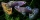Paul had a bowl of guppies for sale. Four customers were milling around the store. 1. Rod told paul - I'll take half the guppies in the bowl, plus had a guppy. 2. Heather said - I'll take half of what you have, plus half a guppy. The third customer, Nancy
• Three unknownsSolve the system of linear equations with three unknowns: A + B + C = 14 B - A - C = 4 2A - B + C = 0
• Stones 3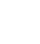Simiyu and Nasike each collected a number of stones in an arithmetic lesson. If Simiyu gave Nasike 5 stones, Nasike would have twice as many stones as Simiyu. If initially, Simiyu had five stones less than Nasike how many stones did each have?
• Age problemsA) Alex is 3 times as old as he was 2 years ago. How old is he now? b) Casey was twice as old as his sister 3 years ago. Now he is 5 years older than his sister. How old is Casey? c) Jessica is 4 years younger than Jennifer now. In 10 years, Jessica will
• Elimination methodSolve system of linear equations by elimination method: 5/2x + 3/5y= 4/15 1/2x + 2/5y= 2/15
• Company and employeesThere are 370 employees in the company - women are 15% less than men. How many men work in the company?
• Flowers 2Cha cruz has a garden. The ratio roses to tulips is 2 : 5, the ratio of roses to orchids is 7 : 6. Cha cruz wonders what the ratio of tulips to orchids is. If Cha cruz has 183 plants, how many of each kind are there?
• Linear function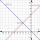What is the equation of linear function passing through points: a) A (0,3), B (3,0) b) A (-2,-6), B (3,4)
• Product V2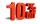The price of product was increased by 12%. Price increased by 273. How much money it would cost this product, if it was on the contrary by 19% discounted.